Learning Library
Who we are
Guidance

# 2.NBT.B.5 Worksheets, Workbooks, Lesson Plans, and Games

#### CCSS.maths.CONTENT.2.NBT.B.5

:
"Fluently add and subtract within 100 using strategies based on place value, properties of operations, and/or the relationship between addition and subtraction."

Our Take:When solving addition and subtraction problems within 100, the year three student can choose from a variety of strategies based on place value and properties of operations. For example, when adding 25 and 46, instead of using the standard method of carrying, the child might focus on place value, breaking both numbers into tens and ones. In this case, the student would find the sum of 20 and 40, then 5 and 6, which adds up to 60 + 11 and ultimately the final answer of 71.

These worksheets, lesson plans, and exercises can help students practise this Common Core State Standards skill.

## Worksheets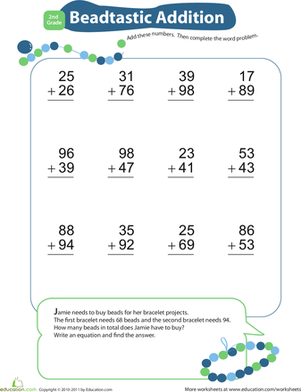Worksheet
This "beadtastic" addition worksheet will get your first grader practising double-digit addition and thinking about some of the real world uses for maths!
Year 3
Maths
Worksheet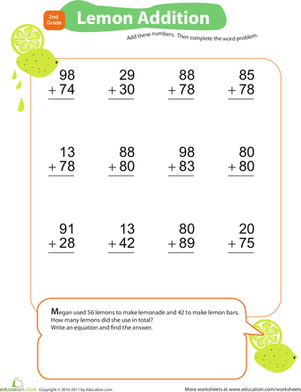Worksheet
This citrus-themed double-digit addition worksheet will spark your first grader's interest in maths!
Year 3
Maths
Worksheet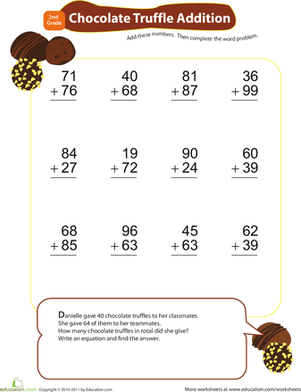Worksheet
If your first grader is gaga over chocolate, enlist her love of the sweet candy to get her interested in double-digit addition!
Year 3
Maths
Worksheet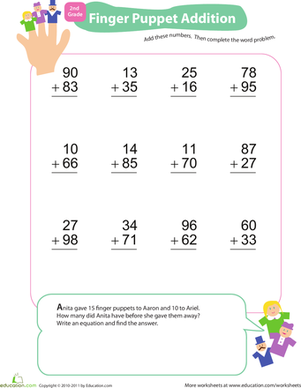Worksheet
This colorful addition worksheet may be finger puppet-themed, but with enough addition practise, your first grader won't be counting fingers!
Year 3
Maths
Worksheet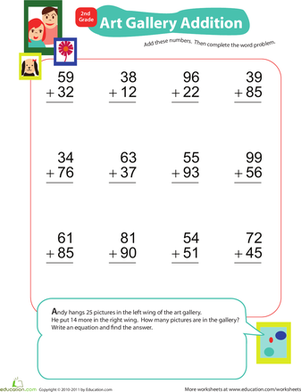Adding Double-Digits at the Art Gallery
Worksheet
Adding Double-Digits at the Art Gallery
This colorful worksheet allows you to combine your second grader's love of art with at-home addition practise with adding double-digits!
Year 3
Maths
Worksheet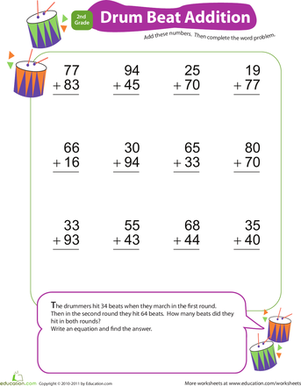Worksheet
Year 3
Maths
Worksheet

## Lesson Plans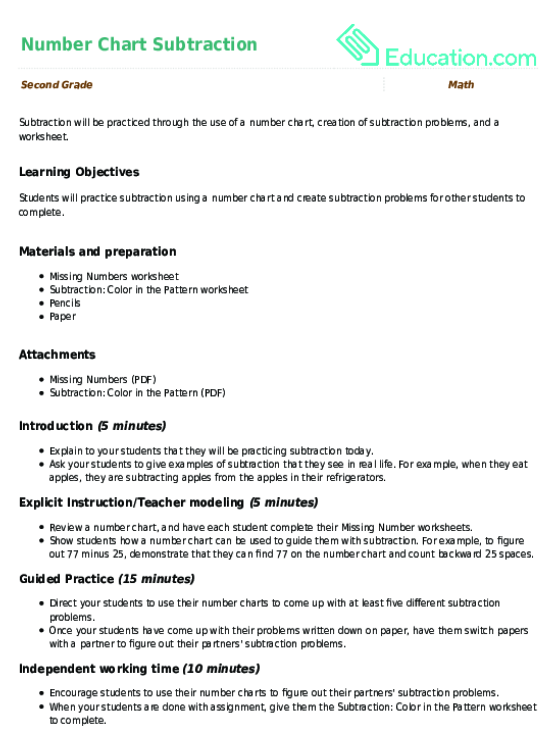Number Chart Subtraction
Lesson plan
Number Chart Subtraction
Subtraction will be practiced through the use of a number chart, creation of subtraction problems, and a worksheet.
Year 3
Maths
Lesson planAdd It Up! Counting Money
Lesson plan
Add It Up! Counting Money
Students will work together as well as individually to add up coins to a desired amount. This real life skill will be made fun when students get to physically count out their cents!
Year 3
Maths
Lesson planYou Be the Judge!
Lesson plan
You Be the Judge!
Give students an opportunity to practise subtraction and analyze their peers' work with this fun lesson. Young mathematicians will have a great time playing a judging game and completing quirky worksheets.
Year 3
Maths
Lesson plan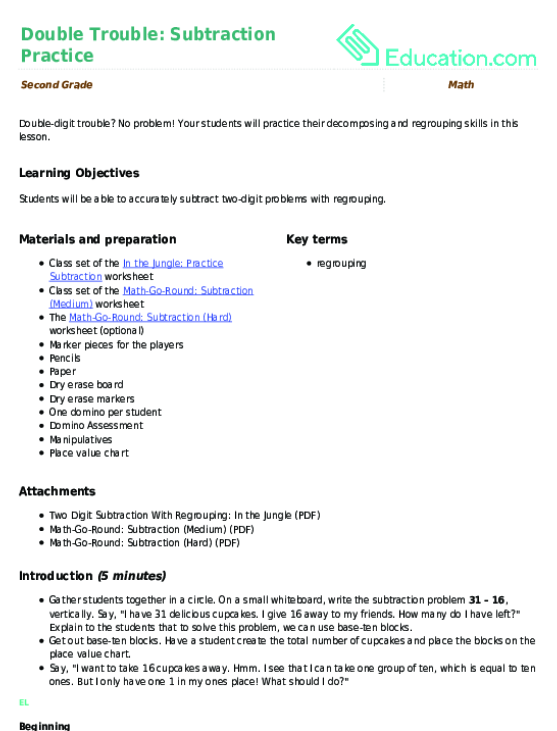Double Trouble: Subtraction practise
Lesson plan
Double Trouble: Subtraction practise
Double-digit trouble? No problem! Your students will practise their decomposing and regrouping skills in this lesson.
Year 3
Maths
Lesson plan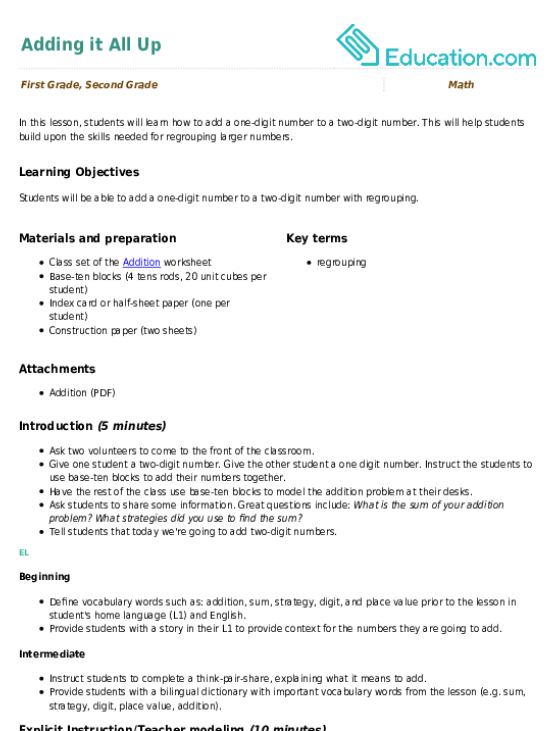Adding it All Up
Lesson plan
Adding it All Up
In this lesson, students will learn how to add a one-digit number to a two-digit number. This will help students build upon the skills needed for regrouping larger numbers.
Year 2
Maths
Lesson planAddition with Regrouping centre
Lesson plan
Addition with Regrouping centre
Make addition with regrouping easy with this lesson plan that makes the task a visual learning experience.
Year 3
Maths
Lesson plan

## Workbooks

No workbooks found for this common core node.

## Games

No games found for this common core node.

## Exercises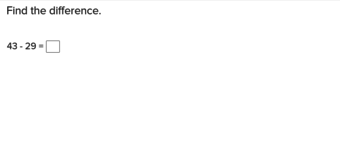Two-Digit Subtraction and Regrouping
Exercise
Two-Digit Subtraction and Regrouping
This exercise showing how to execute two digit subtraction using regrouping makes the second step in subtraction much more accessible.
Year 3
Maths
Exercise

What could we do to improve Education.com?

Please note: Use the Contact Us link at the bottom of our website for account-specific questions or issues.

What would make you love Education.com?